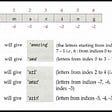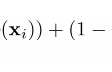# A Simple Embedded Probabilistic Programming Language

What does a dead-simple embedded probabilistic programming language look like? The simplest thing I can imagine involves three components:

• A representation for probabilistic models.
• A way to simulate from those models (‘forward’ sampling).
• A way to sample from a conditional model (‘backward’ sampling).

Rob Zinkov wrote an article on this type of thing around a year ago, and Dan Roy recently gave a talk on the topic as well. In the spirit of unabashed unoriginality, I’ll give a sort of composite example of the two. Most of the material here comes directly from Dan’s talk; definitely check it out if you’re curious about this whole probabilistic programming mumbojumbo.

Let’s whip together a highly-structured, typed, embedded probabilistic programming language — the core of which will encompass only a tiny amount of code.

Some preliminaries — note that you’ll need my simple little mwc-probability library handy for when it comes time to do sampling:

`{-# LANGUAGE DeriveFunctor #-}{-# LANGUAGE LambdaCase #-}import Control.Monadimport Control.Monad.Freeimport qualified System.Random.MWC.Probability as MWC`

# Representing Probabilistic Models

Step one is to represent the fundamental constructs found in probabilistic programs. These are abstract probability distributions; I like to call them models:

`data ModelF r =    BernoulliF Double (Bool -> r)  | BetaF Double Double (Double -> r)  deriving Functortype Model = Free ModelF`

Each foundational probability distribution we want to consider is represented as a constructor of the `ModelF` type. You can think of them as probabilistic instructions, in a sense. A `Model` itself is a program parameterized by this probabilistic instruction set.

In a more sophisticated implementation you’d probably want to add more primitives, but you can get pretty far with the beta and Bernoulli distributions alone. Here are some embedded language terms, only two of which correspond one-to-one with to the constructors themselves:

`bernoulli :: Double -> Model Boolbernoulli p = liftF (BernoulliF p id)beta :: Double -> Double -> Model Doublebeta a b = liftF (BetaF a b id)uniform :: Model Doubleuniform = beta 1 1binomial :: Int -> Double -> Model Intbinomial n p = fmap count coins where  count = length . filter id  coins = replicateM n (bernoulli p)betaBinomial :: Int -> Double -> Double -> Model IntbetaBinomial n a b = do  p <- beta a b  binomial n p`

You can build a lot of other useful distributions by just starting from the beta and Bernoulli as well. And technically I guess the more foundational distributions to use here would be the Dirichlet and categorical, of which the beta and Bernoulli are special cases. But I digress. The point is that other distributions are easy to construct from a set of reliable primitives; you can check out the old lambda-naught paper by Park et al for more examples.

See how `binomial` and `betaBinomial` are defined? In the case of `binomial` we’re using the property that models have a functorial structure by just mapping a counting function over the result of a bunch of Bernoulli random variables. For `betaBinomial` we’re directly making use of our monadic structure, first describing a weight parameter via a beta distribution and then using it as an input to a binomial distribution.

Note in particular that we’ve expressed `betaBinomial` by binding a parameter model to a data model. This is a foundational pattern in Bayesian statistics; in the more usual lingo, the parameter model corresponds to the prior distribution, and the data model is the likelihood.

# Forward-Mode Sampling

So we have our representation. Next up, we want to simulate from these models. Thus far they’re purely abstract, and don’t encode any information about probability or sampling or what have you. We have to ascribe that ourselves.

mwc-probability defines a monadic sampling-based probability distribution type called `Prob`, and we can use a basic recursion scheme on free monads to adapt our own model type to that:

`toSampler :: Model a -> MWC.Prob IO atoSampler = iterM \$ \case  BernoulliF p f -> MWC.bernoulli p >>= f  BetaF a b f    -> MWC.beta a b >>= f`

We can glue that around the relevant mwc-probability functionality to simulate from models directly:

`simulate :: Model a -> IO asimulate model = MWC.withSystemRandom . MWC.asGenIO \$  MWC.sample (toSampler model)`

And this can be used with standard monadic combinators like `replicateM` to collect larger samples:

`> replicateM 10 \$ simulate (betaBinomial 10 1 4)[5,7,1,4,4,1,1,0,4,2]`

# Reverse-Mode Sampling

Now. Here we want to condition our model on some observations and then recover the conditional distribution over its internal parameters.

This part - inference - is what makes probabilistic programming hard, and doing it really well remains an unsolved problem. One of the neat theoretical results in this space due to Ackerman, Freer, and Roy is that in the general case the problem is actually unsolvable, in that one can encode as a probabilistic program a conditional distribution that computes the halting problem. Similarly, in general it’s impossible to do this sort of thing efficiently even for computable conditional distributions. Consider the case of a program that returns the hash of a random n-long binary string, and then try to infer the distribution over strings given some hashes, for example. This is never going to be a tractable problem.

For now let’s use a simple rejection sampler to encode a conditional distribution. We’ll require some observations, a proposal distribution, and the model that we want to invert:

`invert :: (Monad m, Eq b) => m a -> (a -> m b) -> [b] -> m ainvert proposal model observed = loop where  loop = do    parameters <- proposal    generated  <- replicateM (length observed) (model parameters)    if   generated == observed    then return parameters    else loop`

Let’s use it to compute the posterior or inverse model of an (apparently) biased coin, given a few observations. We’ll just use a uniform distribution as our proposal:

`posterior :: Model Doubleposterior = invert [True, True, False, True] uniform bernoulli`

Let’s grab some samples from the posterior distribution:

`> replicateM 1000 (simulate posterior)`

The central tendency of the posterior floats about 0.75, which is what we’d expect, given our observations. This has been inferred from only four points; let’s try adding a few more. But before we do that, note that the present way the rejection sampling algorithm works is:

• Propose a parameter value according to the supplied proposal distribution.
• Generate a sample from the model, of equal size to the supplied observations.
• Compare the collected sample to the supplied observations. If they’re equal, then return the proposed parameter value. Otherwise start over.

Rejection sampling isn’t exactly efficient in nontrivial settings anyway, but it’s supremely inefficient for our present case. The random variables we’re interested in are exchangeable, so what we’re concerned about is the total number of `True` or `False` values observed - not any specific order they appear in.

We can add an ‘assistance’ function to the rejection sampler to help us out in this case:

`invertWithAssistance  :: (Monad m, Eq c)  => ([a] -> c) -> m b -> (b -> m a) -> [a] -> m binvertWithAssistance assister proposal model observed = loop where  len  = length observed  loop = do    parameters <- proposal    generated  <- replicateM len (model parameters)    if   assister generated == assister observed    then return parameters    else loop`

The assister summarizes both our observations and collected sample to ensure they’re efficiently comparable. In our situation, we can use a simple counting function to tally up the number of `True` values we observe:

`count :: [Bool] -> Intcount = length . filter id`

Now let’s create another posterior by conditioning on a few more observations:

`posterior0 :: Model Doubleposterior0 = invertWithAssitance count uniform bernoulli obs where  obs =    [ True, True, True, False    , True, True, False, True    , True, True, True, False    ]`

and collect another thousand samples from it. This would likely take an annoying amount of time without the use of our `count` function for assistance above:

`> replicateM 1000 (simulate posterior0)`

# Conclusion

This is a really basic formulation - too basic to be useful in any meaningful way - but it illustrates some of the most important concepts in probabilistic programming. Representation, simulation, and inference.

I think it’s also particularly nice to do this in Haskell, rather than something like Python (which Dan used in his talk) - it provides us with a lot of extensible structure in a familiar framework for language hacking. It sort of demands you’re a fan of all these higher-kinded types and structured recursions and all that, but if you’re reading this article then you’re probably in that camp anyway.

I’ll probably write a few more little articles like this over time. There are a ton of improvements that we can make to this basic setup - encoding independence, sampling via MCMC, etc. - and it might be fun to grow everything out piece by piece.

I’ve dropped the code from this post into this gist. Credit to Varshesh Joshi for the cover image.

--

--

--

## More from Jared Tobin

Quanty tech guy. https://jtobin.io

Love podcasts or audiobooks? Learn on the go with our new app.## Jared Tobin

Quanty tech guy. https://jtobin.io

## String Slicing And Functions## Intro to NumPy | Why to learn NumPy | NumPy Creating Arrays## Cost Functions## Review of K-Mean Clustering Model